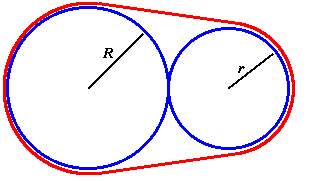#### You may also like### Five Circuits, Seven Spins

A circular plate rolls inside a rectangular tray making five circuits and rotating about its centre seven times. Find the dimensions of the tray.### Approximating Pi

By inscribing a circle in a square and then a square in a circle find an approximation to pi. By using a hexagon, can you improve on the approximation?### A Rolling Disc - Periodic Motion

Imagine a rectangular tray lying flat on a table. Suppose that a plate lies on the tray and rolls around, in contact with the sides as it rolls. What can we say about the motion?

# Belt

##### Age 16 to 18Challenge LevelA length of thin wire ($L$ ) binds together two cylindrical welding rods, whose radii are $R$ and $r$ , by passing all the way around them both. Find $L$ in terms of $R$ and $r$.